### Home > A2C > Chapter 12 > Lesson 12.5.1 > Problem12-195

12-195.
1. Use the Binomial Theorem to write the expansion of each of the following expressions. Homework Help ✎

1.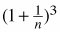2.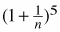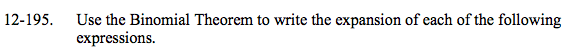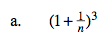$1+\frac{3}{n}+\frac{3}{n^2}+\frac{1}{n^3}$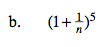Use the same method as in part (a).